Test Description

## 20 Questions MCQ Test NCERT Mathematics for CAT Preparation | Olympiad Test: Linear Equations

Olympiad Test: Linear Equations for Class 8 2022 is part of NCERT Mathematics for CAT Preparation preparation. The Olympiad Test: Linear Equations questions and answers have been prepared according to the Class 8 exam syllabus.The Olympiad Test: Linear Equations MCQs are made for Class 8 2022 Exam. Find important definitions, questions, notes, meanings, examples, exercises, MCQs and online tests for Olympiad Test: Linear Equations below.
Solutions of Olympiad Test: Linear Equations questions in English are available as part of our NCERT Mathematics for CAT Preparation for Class 8 & Olympiad Test: Linear Equations solutions in Hindi for NCERT Mathematics for CAT Preparation course. Download more important topics, notes, lectures and mock test series for Class 8 Exam by signing up for free. Attempt Olympiad Test: Linear Equations | 20 questions in 20 minutes | Mock test for Class 8 preparation | Free important questions MCQ to study NCERT Mathematics for CAT Preparation for Class 8 Exam | Download free PDF with solutions
 1 Crore+ students have signed up on EduRev. Have you?
Olympiad Test: Linear Equations - Question 1

### David cuts a bread into two equal pieces and cuts one half into smaller pieces of equal size. Each of the small pieces is twenty grams in weight. If he has seven pieces of the bread all with him, how heavy is the original cake.

Detailed Solution for Olympiad Test: Linear Equations - Question 1

If she has 7 pieces totally, it means that one of those pieces is the uncut half, and the other half is cut into 6 pieces. Each of those 6 pieces has a weight of 20 gm which totally weighs 6*20= 120gm. If one half is 120 gm, then so is the other. Therefore the total weight of the cake is 120*2= 240 gm.

Olympiad Test: Linear Equations - Question 2

### In the following number sequence, how many such even numbers are there which are exactly divisible by its immediate preceding number but not exactly divisible by its immediate following number? 3  8  4  1  5  7  2  8  3  4  8  9  3  9  4  2  1  5  8  2

Detailed Solution for Olympiad Test: Linear Equations - Question 2

3  8  4  1  5  7  2  8  3  4  8  9  3  9  4  2  1  5  8  2

Olympiad Test: Linear Equations - Question 3

### Mary was counting down from 34 and Thomas was counting upwards simultaneously, the number starting from 1 and he was calling out only the odd numbers. Which common number will they call out at the same time if they were calling out at the same speed?

Detailed Solution for Olympiad Test: Linear Equations - Question 3

Mary = 34,33,32,31,30,29,28,27,26,25,24,23,22
Thomas = 01,03,05,07,09,11,13,15,17,19,21,23,25
Hence they will reach a number 23.

Olympiad Test: Linear Equations - Question 4

Solve the given equation and find the value of y if,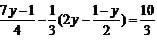Detailed Solution for Olympiad Test: Linear Equations - Question 4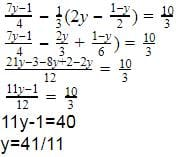Olympiad Test: Linear Equations - Question 5

Find the value of 'm' from the expression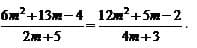Olympiad Test: Linear Equations - Question 6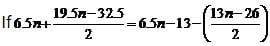then find the value of n.

Olympiad Test: Linear Equations - Question 7

The distance between two mile stones is 230 km and two cars start simultaneously from the milestones in opposite directions and the distance between them after three hours is 20 km. If the speed of one car is less than that of other by 10 km/h, find the speed of each car.

Detailed Solution for Olympiad Test: Linear Equations - Question 7

Let S1 = x and S2 = x+10
Distance = speed * time
D1= 3x, D2 = 3(x+10)
Total distance = D1+D2+20
230 = 3x+30+3x+20
6x = 180
x = 30 km/h
x+10 = 40 km/h

Olympiad Test: Linear Equations - Question 8

A store has provision which would last for a certain number of men for 21 days. For one seventh of the men it will last for how many days?

Olympiad Test: Linear Equations - Question 9

An MNC company employed 25 men to do the official work in 32 days. After 16 days, it employed 5 more men and work was finished one day earlier. If it had not employed additional men, it would have been behind by how many days?

Detailed Solution for Olympiad Test: Linear Equations - Question 9

Let 1 person do 1 unit of work in 1 day
When 25 people are employed, amount of work in 1 day = 25 units
Amount of work in 16 days = 25*13 = 400
After 16 days, 5 more people are employed and work is completed in 15 days
So work done in next 15 days = 30*15 = 450
If those 5 people are not employed , No. of days needed to complete 450 units of work = 450/25 = 18
But they were supposed to complete the work by 16 days
Hence 2 extra days are needed by 25 people.

Olympiad Test: Linear Equations - Question 10

A Bullet train left for the New York at time y minutes after x hours and reached Canada z minutes after y hour on the same day, after travelling z hrs and x minutes. How many possible values of x are there?

Olympiad Test: Linear Equations - Question 11

Solve for x: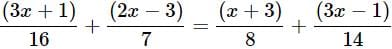Detailed Solution for Olympiad Test: Linear Equations - Question 11

We have,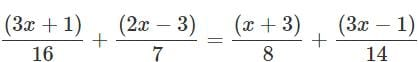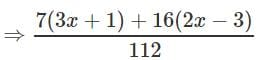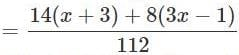⇒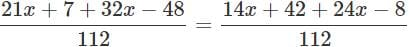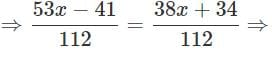553x − 41 = 38x+34 ⇒ 15x = 75

⇒ x = 75/15 = 5

Olympiad Test: Linear Equations - Question 12

A number is 56 greater than the average of its third, quarter and one-twelfth. Find the number.

Detailed Solution for Olympiad Test: Linear Equations - Question 12

Let the number be x. Then, One-third of x = x/3, Quarter of x = x/4
One-twelfth of x = x/12
Average of third, quarter and one-twelfth of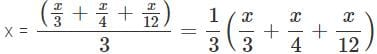According to question, we have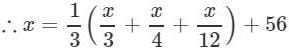⇒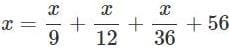⇒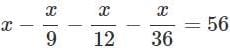⇒ 36x − 4x − 3x − x = 36 × 56 ⇒ 28x = 36 × 56
⇒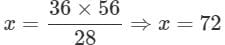Hence, the number is 72.

Olympiad Test: Linear Equations - Question 13

If 1/3 of a number is 10 less than the original number, then the number is_____.

Detailed Solution for Olympiad Test: Linear Equations - Question 13

Let the number bex.
According to question, we have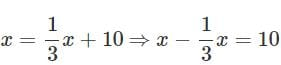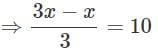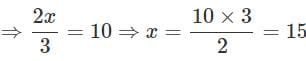Hence, the number is 15.

Olympiad Test: Linear Equations - Question 14

Solve for x : 6(3x+2)−5(6x−1) = 6(x−3)−5(7x−6)+12x

Detailed Solution for Olympiad Test: Linear Equations - Question 14

We have,6(3x+2)−5(6x−1)

= 6(x−3)−5(7x−6)+12x

⇒18x+12−30x+5

= 6x−18−35x+30+12x

⇒ 5x=−5 ⇒ x=−1

Olympiad Test: Linear Equations - Question 15

The number 299 is divided into two parts in the ratio 5:8. The product of the numbers is_____.

Detailed Solution for Olympiad Test: Linear Equations - Question 15

Ratio of two parts = 5:8

Smaller number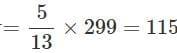Larger number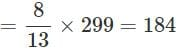So, the product of the number

= 115 × 184 = 21160

Olympiad Test: Linear Equations - Question 16

If (2/3)rd of a number is 20 less than the original number, then the number is_____.

Detailed Solution for Olympiad Test: Linear Equations - Question 16

Let the number be x. According to question, we have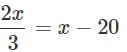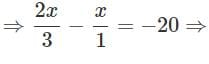Olympiad Test: Linear Equations - Question 17

The perimeter of a rectangle is numerically equal to the area of rectangle. If width of rectangle is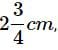then its length is_____.

Detailed Solution for Olympiad Test: Linear Equations - Question 17

Let the length of rectangle be x cm. Width of rectangle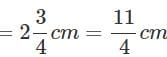∴ Area of rectangle = length × width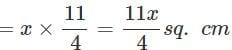Also, perimeter of rectangle = 2(length + width) Now, according to question.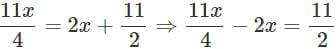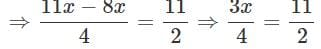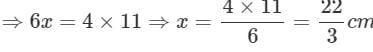Olympiad Test: Linear Equations - Question 18

A number whose seventh part exceeds its eighth part by 1, is _____.

Detailed Solution for Olympiad Test: Linear Equations - Question 18

Let the number be x. According to question, we have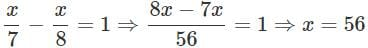Olympiad Test: Linear Equations - Question 19

A number consists of two digits whose sum is 9. If 27 is subtracted from the original number, its digits are interchanged. Then the original number is _____.

Detailed Solution for Olympiad Test: Linear Equations - Question 19

Let units place digit be x. So tens place digit = 9 − x.

∴ The original number = 10(9-x)+x = 90-10x + x=90 - 9x Now, after interchanging the digits, the number formed = 10 (x) + 9 - x = 9x + 9

∴ According to question, we have 90 - 9x - 27 = 9x + 9

⇒ −18x = −54 ⇒ x = 3

So, the original number

= 90−9×3 = 63

Olympiad Test: Linear Equations - Question 20

The denominator of a rational number is greater than its numerator by 3. If 3 is subtracted from the numerator and 2 is added to its denominator, the new number becomes 1/5. The original rational number is;___.

Detailed Solution for Olympiad Test: Linear Equations - Question 20

Let numerator be x, then denominator is x+3.

∴ The original rational number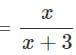Now, according to question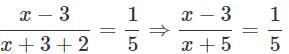On cross multiplying, we get  5(x−3 )= x+5

⇒ 5x−15 = x+5 ⇒ 5x−x = 5+15

⇒ 4x = 20 ⇒ x = 20/4 = 5

Hence, the original rational number is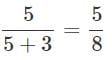## NCERT Mathematics for CAT Preparation

276 docs|149 tests
 Use Code STAYHOME200 and get INR 200 additional OFF Use Coupon Code